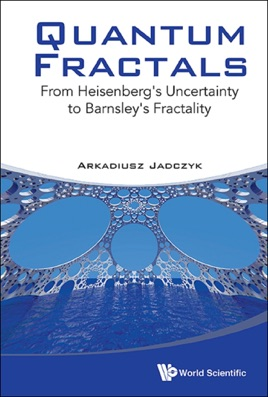• USD 43.99

## Descripción de editorial

Starting with numerical algorithms resulting in new kinds of amazing fractal patterns on the sphere, this book describes the theory underlying these phenomena and indicates possible future applications. The book also explores the following questions:
What are fractals?How do fractal patterns emerge from quantum observations and relativistic light aberration effects?What are the open problems with iterated function systems based on Mobius transformations?Can quantum fractals be experimentally detected?What are quantum jumps?Is quantum theory complete and/or universal?Is the standard interpretation of Heisenberg's uncertainty relations accurate?What is Event Enhanced Quantum Theory and how does it differ from spontaneous localization theories?What are the possible applications of quantum fractals? Contents:IntroductionWhat are Quantum Fractals?:Cantor SetIterated Function SystemsCantor Set Through Matrix EigenvectorQuantum Iterated Function SystemsExample: The "Impossible" Quantum FractalAction on the PlaneLorentz Group, SL(2,ℂ), and Relativistic AberrationExamples:Hyperbolic Quantum FractalsControlling Chaotic Behavior and Fractal DimensionQuantum Fractals on n-SpheresAlgorithms for Generating Hyperbolic Quantum FractalsFoundational Questions:Stochastic Nature of Quantum Measurement ProcessesAre There Quantum Jumps?Bohmian MechanicsEvent Enhanced Quantum TheoryGhirardi–Rimini–Weber Spontaneous LocalizationHeisenberg's Uncertainty Principle and Quantum FractalsAre Quantum Fractals Real?Appendix A. Mathematical Concepts:Metric SpacesNormed SpacesMeasure and IntegralMarkov, Frobenius-Perron and Koopman OperatorsAppendix B. Minkowski Space Generalization of Euler-Rodrigues Formula:Alternative Derivation via SL(2,ℂ)